# Prime Numbers

## Mathematician Gareth Jones on number theory, why there are infinitely many of prime numbers and how to calculate the proportion of prime numbers up to any given n

### videos | March 17, 2020

I’d like to tell you about prime numbers. These arise in an area of mathematics called ‘number theory’. One can trace their history back more than two millennia to the ancient Greeks, but they are still of fundamental importance. For instance, their properties are used in the cryptographic systems that we use to ensure the safety and security of electronic communications.

What is a prime number? Well, it’s an integer greater than 1 which is divisible only by 1 and by itself, only by the obvious factors. So for example, 2, 3, 5 and 7 are prime numbers, but 4, 6, 8 are not, they’re all divisible by 2. In a sense, they form the building blocks of number theory. This is explained by what’s called the fundamental theorem of arithmetic which states that any integer greater than 1 can be written in an essentially unique way as a product of finitely many prime numbers. So for instance, 6 is 2*3, a product of two primes. 20 is 2*2*5. In this finite product we allow just one factor, so for instance, 5 is the one factor, it is prime. So each integer greater than 1 then can be decomposed into prime numbers. I said ‘in an essentially unique way’: what do I mean by that? Well, we can write the factors in any order: I can write 6 = 3 *2. In general, I can write the factors in any order I like but that is the only freedom I have: the same factors must be used.

One important property of prime numbers is that there are infinitely many of them. This is a theorem that goes right back to Euclid, it’s in his Elements. I’ll outline Euclid’s proof of this for you. Suppose, we take any finite set of prime numbers: I can use that to produce a new prime number that’s not in that set. Let’s take the finite set of prime numbers and multiply them all together and call that integer n. So all the prime numbers we chose divide n. Now look at n+1, apply the fundamental theorem of arithmetic to that. It’s a product of prime numbers, so there must be some prime number which divides it. That prime number cannot be one of the finite set we started with, because if it was, it would divide n and it would divide n+1, so it would have to divide their difference which is 1, but 1 is not divisible by any prime number, so we have produced a new prime number. For instance, if we start with 2, 3 and 5, and multiply them together, we get 30; add 1, we get 31. That is a new prime number. And you will always get a new prime factor by this process. So there cannot be only finitely many primes; we can always produce a new one, so there must be infinitely many primes.

Now, when you look at the distribution of these primes something rather strange happens as you go along the number system, picking out the prime numbers: there’s no regular pattern but they seem to become less and less frequent as the numbers become larger. For example, there are 4 primes less than 10, there are 25 primes less than 100, and there are 168 primes less than 1000. So the proportion of primes up to 10 is 0.4, up to 100 it’s 0.25 and up to 1000 it’s 0.168. The proportion of primes is going down as the numbers increase even though there are infinitely many primes.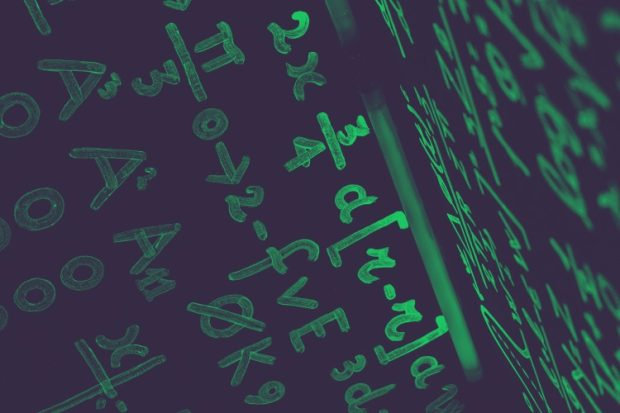Mathematician and Historian of Mathematics Jeremy Gray on the theory of quadratic forms, the rediscovery of Ceres, and non-Euclidian geometry
In the 1790s Gauss, who was still a schoolboy at the time, produced huge tables of prime numbers and he counted them just as I’ve outlined. What he noticed was that the proportion of primes up to a given integer n is approximately 1 over the natural logarithm of n (1/ln n). Now, you don’t need to know what the natural logarithm function is but it increases as n increases, but it increases more and more slowly: it’s roughly twice the number of decimal digits in n if you want to know roughly how big it is. What it means is that the proportion of integers which are prime up to n is slowly, very slowly going towards zero. And Gauss conjectured that this would be true for all integers n, not just the ones he had been able to calculate.

He couldn’t prove it but in 1896 two mathematicians, Hadamard and de la Vallée Poussin, independently proved it. This is what’s called the ‘prime number theorem’: it says that for all integers n the proportion of numbers up to n which are prime is approximately 1/ln n. So it tells us how fast the prime numbers are thinning out as we go up into larger and larger numbers. To put it another way, the average gap between a prime number p and the next prime number is about the ln p. It could be very small, it could be just 2, it could be arbitrarily large, but on average it’s about the logarithm of p.

It’s not an exact estimate: I said ‘approximately 1/ln n’, and what we would really like to know is how good an approximation that is. We have some information, but we would like to have more precise information. Riemann in the middle of the 19th century tried to do this; he used what is now called the ‘Riemann zeta-function’. This was actually introduced by Euler in the 1730s. What Euler proved was that this function can be written as a product of infinitely many factors, one for each prime number, and it’s this connection with prime numbers which means that understanding the behaviour of this function gives us some insight into the distribution of prime numbers. Riemann extended the definition of this function from real numbers to complex numbers and using this extra power he was able to connect the distribution of prime numbers with the zeros of this function. These are the complex numbers where the function takes the value zero.

We know infinitely many of these complex numbers. They are the negative even integers and certain complex numbers with real part equal to a half. We conjecture that these are the only zeros, that is what Riemann conjectured.

That is called the Riemann hypothesis: that the zeros of this function are just the ones that we know about, there aren’t any unexpected zeros that we haven’t yet found.

If that could be proved it would give us much more information about how accurate 1/ln n is for the proportion of primes between 1 and n and it would also help us with many other similar estimates in number theory. It was conjectured 160 years ago by Riemann, and many mathematicians have tried to prove it since then, and it is still open, it is widely regarded as one of the most important and prestigious open problems in mathematics.

In 2000 the Clay Mathematics Institute proposed seven prizes for mathematicians to solve, each of which was worth a million dollars. One of them was proved within a couple of years: a Russian mathematician Grigori Perelman proved the Poincaré conjecture. Perlman is a very modest and reclusive character: he declined the cash prize and he declined the fields medal that he was awarded soon afterwards. It was perhaps the greatest achievement so far of 21st-century mathematics. The remaining six problems, including the Riemann hypothesis, are still open, so if you’re feeling short of money at any time, if you feel you need another million dollars in your bank account, there is the opportunity: go and prove the Riemann hypothesis. But I warn you: it will not be easy.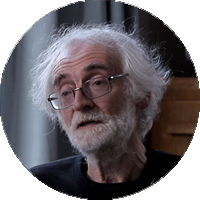Emeritus Professor of Mathematics, University of Southampton
Did you like it? Share it with your friends!
Published items
0779
To be published soon
+92

#### Most viewed

• 1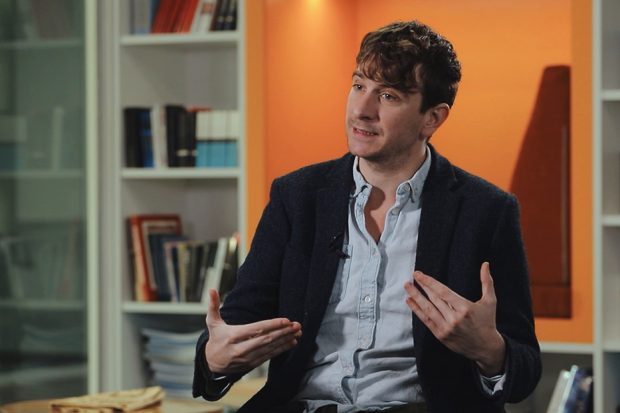Peter Jones
• 2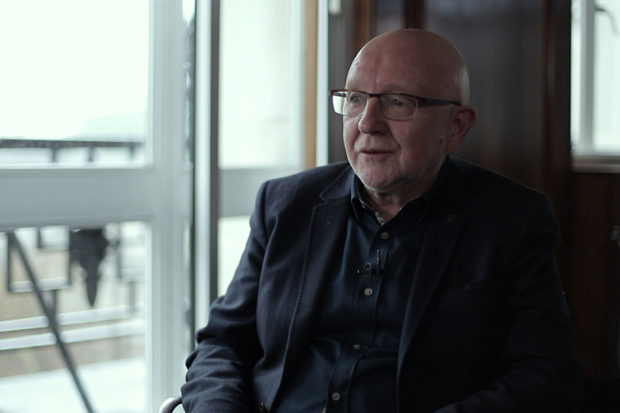John Worrall
• 3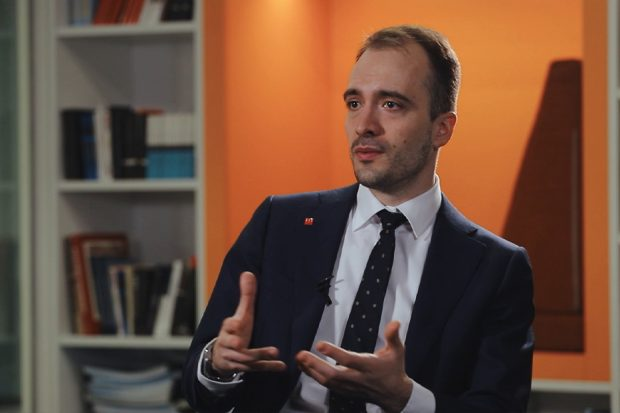Daniel Kontowski
• 4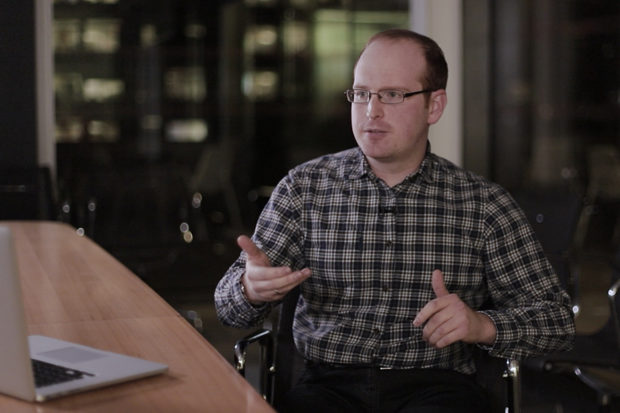Jonathan Birch
• 5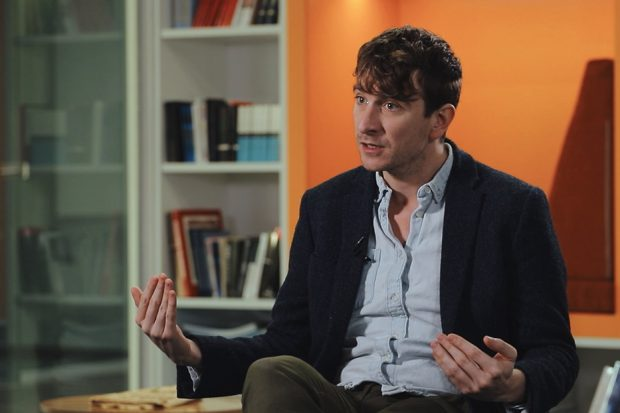Peter Jones
• 6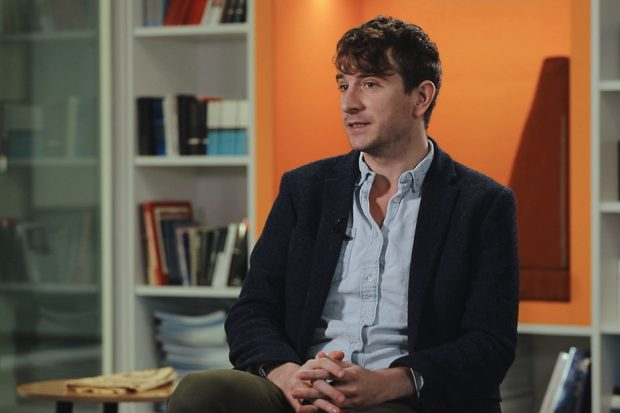Peter Jones
• 7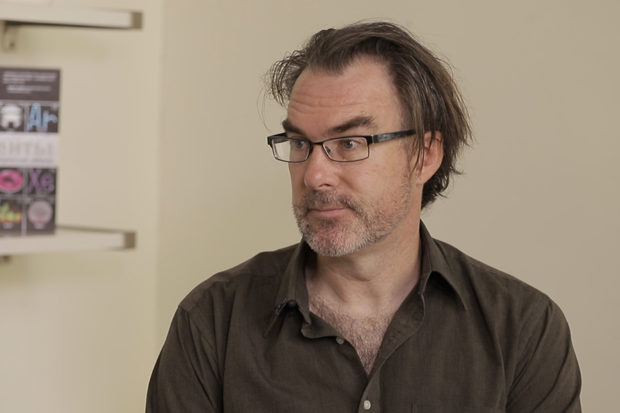Jeffrey Taylor
• 8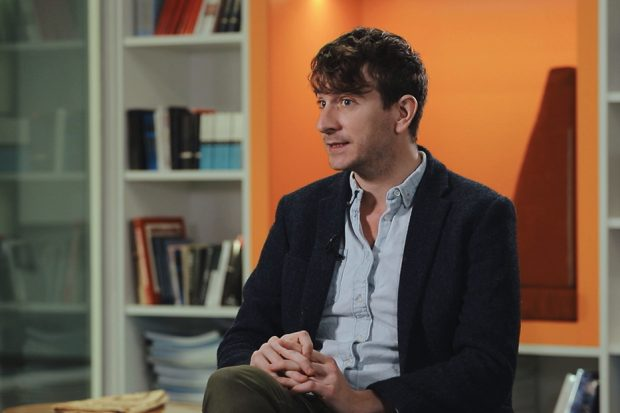Peter Jones
• 9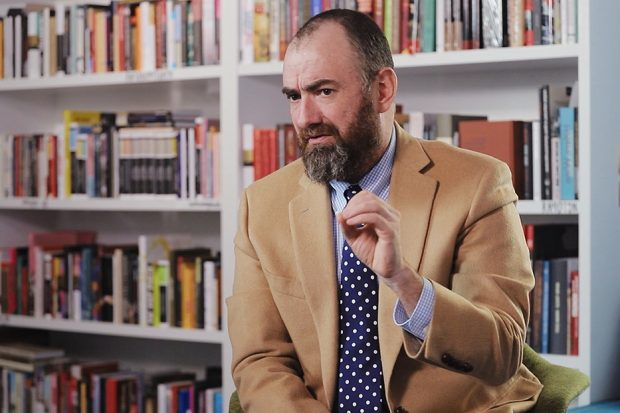David Dussault
•